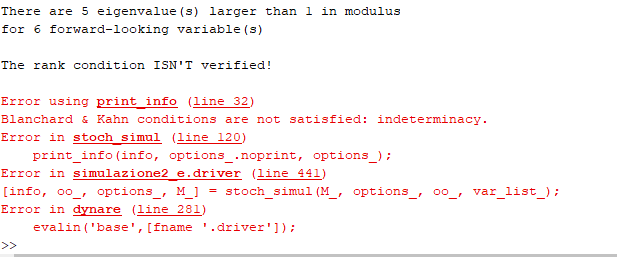# Error: the following equations have non-zero second derivatives

Hello everyone, i try to run these code, but there is a problem whit the equation number 2 and 9/10. In particular, there is a term, X, that is neither a variable a parameters, but if I replace it in the equation, the number of equations and variables no longer coincides and I don’t know where to insert it. Can someone take a look at this code, especially the equations? Since the errors reported by the code are:

ERROR: If the model is declared linear the second derivatives must be equal to zero.
The following equations have non-zero second derivatives:
* Eq # 2 [c]
* Eq # 9 

Thanks so much for your help

simulazione_e.mod (3.3 KB)

The exponential function in

``````c=exp(c(+1))-(i-exp(pic(+1)));
``````

is clearly not a linear function.

dear professor, thank you for replying. I think the problem is due precisely to the time setting, in particular the equations that cause problems have the expected value inside, but by defining it with (+1), it does not change.simulazione2_e.mod (2.6 KB)

`exp` is the exponential function, not expectations. The expectations are taken automatically by Dynare if there is a `(1)` or `(+1)` in the timing.

Dear professor, sorry to bother you again and thank you for your precious help. I solved most of the problems thanks to your advice. But I have another question; in the reference paper they introduce an equation, X, used as mathematical adjustments, but X is neither a variable nor a parameter, however it is inserted between the equations. I was wondering where I had to define it in the code, because if I don’t insert it the number of variables and equations no longer returns. I try to put it in the steady state but in these way appears this error:simulazione2_e.mod (2.5 KB)

`X` is an auxiliary variable with its own equation. That is correct. But you are missing
``````predetermined_variables k;